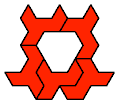# Polyomino and Polyking Tiling

## Tiling Rectangles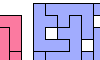Polyomino Rectification with Holes. Tile a rectangle with a given polyomino, allowing isolated one-cell holes.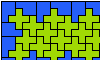Two-Pentomino Balanced Rectangles. Tile a rectangle with two pentominoes in equal quantities.Two-Pentomino Holey Balanced Rectangles. Tile a rectangle with two pentominoes in equal quantities, allowing one-cell holes.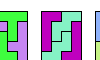Scaled Two-Pentomino Rectangles. Tile a rectangle with two pentominoes at various sizes.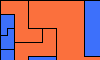Scaled Two-Pentomino Balanced Rectangles. Tile a rectangle with various sizes of two pentominoes in equal areas.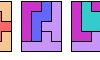Three-Pentomino Rectangles. Tile a rectangle with copies of three pentominoes.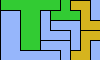Scaled Three-Pentomino Rectangles. Tile a rectangle with three pentominoes at various sizes.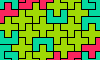Three-Pentomino Balanced Rectangles. Tile a rectangle with three pentominoes in equal quantities.Three-Pentomino Holey Balanced Rectangles. Tile a rectangle with three pentominoes in equal quantities, allowing one-cell holes.Scaled Three-Pentomino Balanced Rectangles. Tile a rectangle with various sizes of three pentominoes in equal areas.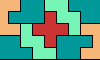Separated Pentominoes Tiling a Rectangle. Tile the largest possible rectangle with copies of three or four pentominoes, with no two copies of the same pentomino touching.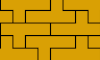Prime Rectangle Tilings for the Y Pentomino. Irreducible rectangles formed of Y pentominoes.Yin-Yang Dominoes. Arrange 10 of the 12 pentominoes to cover a bi-colored domino.Hexomino Pair Rectangles. Arrange copies of two hexominoes to form a rectangle.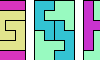Scaled Hexomino Pair Rectangles. Arrange copies of two hexominoes at various scales to form a rectangle.Prime Rectangles for Tetrakings.. For each tetraking, find the irreducible rectangles that it can tile.

## Tiling L-Shaped Polyominoes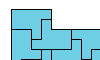Tiling an L Shape with a Polyomino. Tile an L-shaped polyomino with copies of a given polyomino.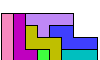Tiling an L Shape with the 12 Pentominoes. Tile various L-shaped polyominoes with the 12 pentominoes.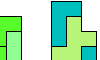Tiling an L Shape with a Tetromino and a Pentomino. Tile an L-shaped polyomino with copies of a given tetromino and pentomino.L Shapes From Two Pentominoes. Form an L-shaped (hexagonal) polyomino with copies of two pentominoes, using at least one of each.Holey L Shapes From Two Pentominoes. Form an L-shaped (hexagonal) polyomino with copies of two pentominoes, using at least one of each, and allowing one-celled holes that do not touch the perimeter or one another.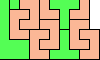Scaled Two-Pentomino L Shapes. Form an L-shaped (hexagonal) polyomino with copies of two pentominoes, letting them be enlarged, using at least one of each.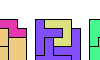L Shapes From Two Hexominoes. Form an L-shaped (hexagonal) polyomino with copies of two hexominoes, using at least one of each.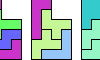Tiling an L Shape with Three Pentominoes. Tile an L-shaped polyomino with copies of three given pentominoes.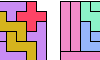Scaled Three-Pentomino L Shapes. Tile an L-shaped polyomino with copies of three given pentominoes at various sizes.

## Other Tilings and Coverings

Back to Polyform Tiling < Polyform Curiosities
Col. George Sicherman [ HOME | MAIL ]